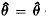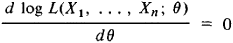# Maximum Likelihood Method

(redirected from Maximum likelihood)
Also found in: Dictionary, Medical, Acronyms, Wikipedia.

## maximum likelihood method

[′mak·sə·məm ′līk·lē‚hu̇d ‚meth·əd]
(statistics)
A technique in statistics where the likelihood distribution is so maximized as to produce an estimate to the random variables involved.
McGraw-Hill Dictionary of Scientific & Technical Terms, 6E, Copyright © 2003 by The McGraw-Hill Companies, Inc.
The following article is from The Great Soviet Encyclopedia (1979). It might be outdated or ideologically biased.

## Maximum Likelihood Method

a method of finding statistical estimates of the unknown parameters of a distribution. According to the maximum likelihood method, we select as the estimates of the parameters those values for which the data resulting from observations are “most likely.” It is assumed that the results of observations X1, …, Xn are mutually independent random variables with identical probability distributions, all depending on the same unknown parameter θ ε ө, where ө is the set of admissible values of θ. To assign an exact meaning to the concept of “most likely,” we proceed by introducing a function

L(x1, …, xn; θ) = p(x1; θ) … p(xn; θ)

where p(t;θ) for a continuous distribution is interpreted as the probability density of the random variable X, and in the discrete case as the probability that the random variable X takes the value t. The function L(X1, …, Xn;θ) of the random variables X1,…, Xn is called the likelihood function, and the maximum likelihood estimate of the parameter θ is that value(X1, …, Xn) (which is itself a random variable) of θ for which the likelihood function attains the largest possible value. Since the maximum point for log L is the same as that for L, it is usually sufficient to solve the so-called likelihood equationin order to find the maximum likelihood estimates.

The maximum likelihood method does not always lead to acceptable results but in some sense is the best method for a broad set of cases of practical importance. For example, we may assert that if there exists an efficient unbiased estimate θ* for the parameter θ in a sample of size n, then the likelihood equation will have the unique solution θ = θ*. In dealing with the asymptotic behavior of maximum likelihood estimates for large n, it is well known that the maximum likelihood method leads under certain general conditions to a consistent estimate that is asymptotically normal and asymptotically efficient. The definitions given above can be generalized to the case of several unknown parameters and to the case of samples from multivariate distributions.

The maximum likelihood method in its modern form was proposed by the British statistician R. Fisher in 1912, although particular forms of the method were used by K. Gauss; even earlier, in the 18th century, J. Lambert and D. Bernoulli came close to the idea of the method.

### REFERENCES

Cramer, H. Matematicheskie metody slalisliki. Moscow, 1948. (Translated from English.)
Rao, C. R. Lineinye statisticheskie metody i ikh primeneniia. Moscow, 1968. (Translated from English.)
Hudson, D. Statistika dlia fizikov. Moscow, 1970. (Translated from English.)

A. V. PROKHOROV

References in periodicals archive ?
The maximum likelihood method is used to develop estimators for the parameters of the new family.
Maximum likelihood estimation using price data of the derivative contract.
The modified maximum likelihood method, maximum likelihood method, energy pattern factor method, empirical method and method of moment are applied to calculate parameters 'k and 'c'.
Many techniques exist for estimation, among which some of the most well-known techniques include Maximum Likelihood (ML) estimation, Method of Moments (MM) and Decision-Directed (DD) method.
To avoid overwhelming the reader with mathematical details, we present the supporting derivations in the "Derivation of the Procedure for Parameter Estimation" and "Equivalence of Maximum Likelihood and Method of Moments Estimates" sections.
The maximum likelihood estimation is different from other estimation methods.
It is a triparametric distribution (location, scale, and shape) and parameter estimation via maximum likelihood and the method of moments have been reported in , concluding that the estimates do not have a closed form and must be obtained numerically.
After the coordinates of the unknown node are estimated with the maximum likelihood estimation method, the parameters in the radio signal propagation model, [mathematical expression not reproducible], are estimated using the least square method.
The book has 12 chapters; the first two deal with models of nucleotide, amino acid, and codon substitution models, which are important for maximum likelihood and Bayesian methods of reconstruction.
This paper discusses the unsupervised clustering practice of largely distributed data by utilizing Expectation Maximization (EM) and Maximum Likelihood (ML) in Gaussian Mixture Model.

Site: Follow: Share:
Open / Close